If you have a related question, please click the "Ask a related question" button in the top right corner. The newly created question will be automatically linked to this question.

# OPA858: Transimpedance Amplifier Output Oscillation

Part Number: OPA858
Other Parts Discussed in Thread: TINA-TI, , TPS61391, OPA356, OPA695, OPA859

Hello all,

I have designed a transimpedance amplifier to detect a pulse signal received by a photodiode. V_APD is the APD supply, V_COM is the DC voltage that will create an offset at the output and PD is the signal that enables/disables the Op Amp. The circuit schematic is below.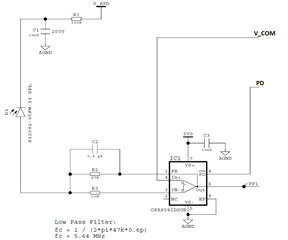After the Op Amp is supplied, I connect the DC voltage of 2V from a power supply to V_COM and measure it at the oscilloscope (green waveform). However, when I connect a probe to the Op Amp output (yellow waveform) both signals start to oscillate. You can see this behaviour on the screen below. Why is this happening? Is the probe capacitance making the circuit unstable? How should I measure the signal?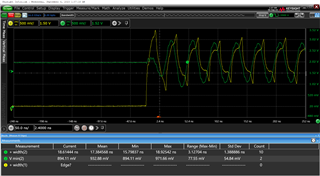João Rego

• Hi João,

I simulated the circuit you modeled and also completed a stability analysis. My observations:

• The circuit you propose is stable because it has a phase margin of 54 degrees
• Adding capacitance of 5pF to the output to simulate the capacitance of the oscilloscope probe changes the phase margin to 38 degrees, making the circuit marginally stable and prone to oscillations. The phase margin would drop even lower if the probe capacitance is greater than 5pF

My suggestions:

• To measure the signal, you should add an isolation resistor to increase stability at the cost of a slight voltage drop on the output. You can learn more about isolation resistors at the TI Precison Lab Op Amp Video Series under the stability section, the video on isolation resistors.
• You could also try to measure the signal, if possible, using 50R cabling that is terminated on both ends so that there is not large added capacitance to the output
• The stability analysis I completed also does not account for any stray PCB capacitance. If you have an estimate of the stray PCB capacitance, I recommend including that to get a more accurate result. I have included the stability analysis TINA-TI circuit so you can make adjustments as needed.

Please let me know if you have any other questions.

OPA858stability_E2E.TSC

Thanks,

Nick

• Hi Nick,

Thank you for your response and for sharing the simulation.

I saw your simulation and it makes sense why the circuit is oscillating. The probe has a capacitance of around 10-25pF, so the phase margin is even lower, as you said. This leads to an output oscillation.

I will add an isolation resistor to decrease the effect of the probe capacitive loading. A 1kOhm resistor should be enough since it makes the phase margin 54 degrees, as the circuit was designed.

Although I have a BNC connector (to measure signals at 50Ohm impedance) at the PCB, when I tried to use it, the oscillation still occurred because I had to solder a cable from the TIA output to the connector. Of course, this increased the output capacitance.

Can you help me with another question related to this topic? At first, I had the circuit below. In the 1st stage, a transimpedance amplifier is followed by an inverting amplifier in the 2nd stage with a gain of 100 and the same offset as the 1st stage. This 2nd stage also has a pass band filter. Both have the same connection to the non-inverting input (V_COM) which is generated outside the PCB through the power supply or a resistor divider. For these tests, I used a resistor divider of 10kOhm and 22kOhm connected to 3V at the power supply. I also had a capacitor of 2.2uF after the resistor divider to remove the noise from the power supply.When I turned on the circuit, it was oscillating at the beginning until the Op Amps reached saturation. The waveforms are below, where the yellow triangular waveform is the V_COM pin, the green waveform is the 2nd stage output (measured with a 50Ohm cable) and the rectangular yellow waveform is the 1st stage output (measured with a 10MOhm probe). At first, I thought the problem was the use of the same connection for both Op Amp’s non-inverting inputs. That’s why I removed the 2nd stage and tested the 1st stage alone. Now, I believe that it is something related to instability in the 2nd stage. What do you think?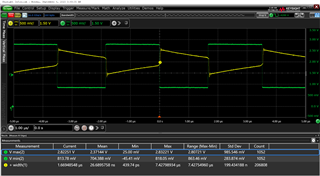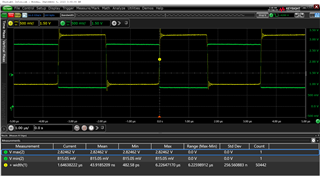Thanks,

João Rego

• Hi João,

I ran a stability analysis of the second stage (inverting amplifier) and that stage has a phase margin about 65 degrees so it is not the stability of the second stage exactly. I believe the reason you are seeing the V_COM behaving as a triangular waveform instead of a steady DC voltage is that you are overdriving the second stage amplifier. Since you have such a large gain, the output is getting saturated at the output swing limit and the inverting terminal will keep trying to match that value which will pull the non-inverting terminal up to match it. My recommendation is to adjust the gain so that you are not heavily overdriving the opamp, since it appears there is not a need for such a large gain on the second stage.

Thanks,

Nick

• Hi Nick,

I added the 1kOhm isolation resistor to the output of the 1st stage (TIA) and the signal at the oscilloscope was still oscillating (image below). However, in this case, only the output of the TIA was oscillating (yellow waveform), while the V_COM signal was a steady DC voltage. Then, I used a 10kOhm isolation resistor at the output and the oscillation was gone. This 10kOhm resistor made the TIA stable as well as working as expected when the APD received a signal. My question is, how can I design this isolation resistor value? Although, the system was stable in simulation with the 1kOhm resistor, in the laboratory didn't work.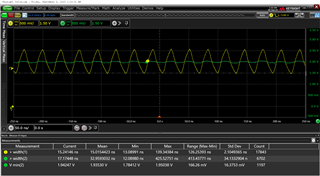Regarding the second topic, I decreased the 2nd stage gain to 1 (replaced the 10kOhm resistor with 100Ohm). Similar to the previous circuit, the oscillation at V_COM was gone, however, there was an oscillation at the 2nd stage output. This signal was measured with a 50Ohm probe. When I used a 1MOhm probe, the oscillation disappeared and the circuit was working well. Do you know why there is an oscillation with the 50Ohm probe?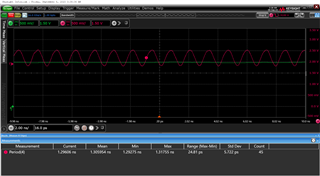I also changed the feedback resistor to 1kOhm, to have a gain of 10, and the circuit was also operational.

I hope I did explain my problem clearly.

Thank you,

João Rego

• Hi João,

Regarding your measurements of the first stage, the stability analysis simulation that I sent you did not account for stray PCB capacitance and therefore is not an exact simulation for the real-world device. Especially since the OPA858 operates at a very high speed, it is not nearly as forgiving in design or measurement. It is very likely that while 1kohm provided enough stability in the simulation, it was not enough to overcome effects in the lab.

Regarding your measurements of the second stage, I believe the reason you are seeing oscillations when you measure with the 50ohm probe is because the OPA858 is not a unity gain stable part, so setting the amplifier to a gain of 1 causes the circuit to become unstable. Using the 1Mohm probe likely heavily damped the output, so the oscillations were not clear. But looking closely at the 1Mohm probe output, it looks like there might still be some oscillations happening just without high peaks.

I am assuming that setting the gain to 10 and measuring the output with the 50 ohm probe you are not seeing oscillations and the circuit is operating as it should. Please correct me if I have that incorrect and I will help determine if any other issues are arising.

Thanks,

Nick

• Hi Nick,

I tested the gain of 10 (feedback resistor =1kOhm) and the oscillation still occurred with the 50Ohm probe. I also added a series resistor of 47 Ohm and the oscillation was still present. Below is the 2nd stage output (pink) and the offset signal on the IN+ input (green).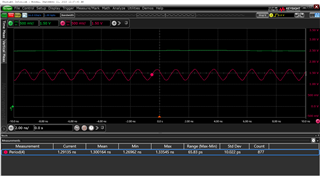When I added the 1MOhm probe, the oscillation almost disappeared. In fact, there is some oscillation but it is negligible. The waveforms are below (yellow: 2nd stage output; green: IN+ input). However, as you can see, the 2nd stage output voltage isn't equal to the IN+ input. Why is this happening? Shouldn't they be the same when no signal is coming from the TIA?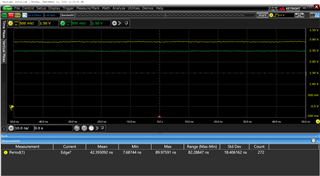Then, I increased the gain again to 100 and the circuit had the same problems as the one with the gain of 10. However, the initial problem was solved (oscillation). Below is the schematic, where the 1pF capacitor on the 2nd stage was removed and the 1nF capacitor was added to the connector connected to the IN+ inputs. Currently, I have two problems with this circuit: I can only measure with a 1MOhm probe since the 50Ohm introduces oscillation; and the 2nd stage output doesn't follow correctly the IN+ input.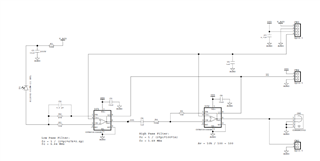The IN+ input signal is generated through the Op Amp supply and a resistor divider of 10kOhm and 22kOhm (22k/(22k+10k)). In this case, with an Op Amp supplied by 4V, the IN+ input should be 2.6V. However, the 2nd stage output has a lower value than this. Why?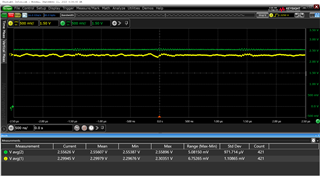Then, I supplied the resistor divider with a voltage that didn't come from the Op Amp supply. I used 3V from another channel of the power supply and connected it to the resistor divider. This voltage should be 2V, however, in the scope is 2.5V. Also, the 2nd stage output has a higher voltage than the IN+ input.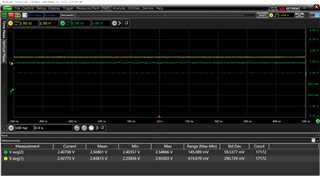When I removed the resistor divider and connected a 2V DC value from the power supply directly to the IN+ pin, I got an oscillation on the 2nd stage output. This was the most strange behaviour.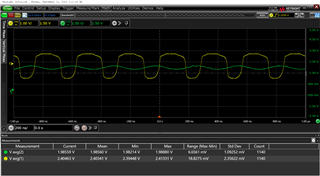In recap, why can't I measure the output signal with a 50Ohm probe? And why does the IN+ input signal as this strange behaviour, where depending on the reference it has an unpredictable action?

Thanks,

João Rego

• Hi João,

I want to confirm a few things:

1. Is this the configuration that you are testing?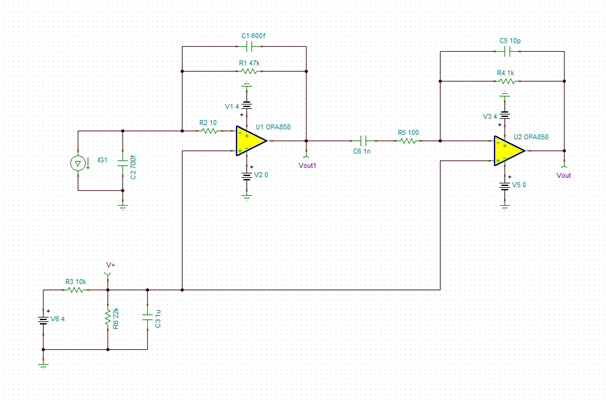You have talked about changing a few different things in testing and I want to make sure that we are talking about the same circuit. Please let me know if anything is missing or different than what you are testing.
2. When you are testing, is the photodiode providing an input signal? Or are you measuring the second stage with the first stage disconnected?
3. It is possible that 50 ohm probe is showing oscillations because it is not working properly. Can you confirm whether it is a probe issue by trying a different 50 ohm probe? Also, the 1Mohm probe will not show the power reflections from a impedance mismatch because you are not testing an AC signal currently.
4. Are you still including the isolation resistor after the first stage that connects with the second stage when testing the output of the second stage?

One thing to note:

The OPA858 has a common-mode input range that is 0V to 1.4V less than the positive supply voltage (Vs+). So if you are supplying 4V, the max common-mode input voltage is 2.6V. When the voltage divider was powered with the 4V op amp supply, it actually outputs 2.75V which will cause unexpected behavior from exceeding the limits.

Thanks,

Nick

• Hi Nick,

1. Currently, I am testing this circuit. In the lab, I noticed that removing the 1pF capacitor in the 2nd stage, allowed me to use the gain of 100. So, I swapped the 1kOhm resistor for the 10kOhm.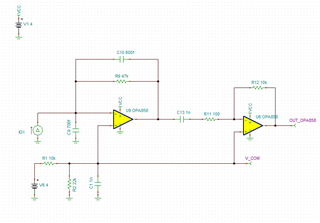2. No, the photodiode isn't providing an input signal. I measured the TIA IN+ pin and the TIA output (1st stage) and they were equal as expected. So, I am measuring the 2nd stage with the 1st stage connected. I can mention that although the signals IN+ and the 2nd stage output don't have the same DC value, when I provide a signal to the photodiode, the 2nd stage output reacts to that signal. That's why I say that the circuit is working.

3. I tested with another 50Ohm probe and the oscillations were still there.

4. After I tested the first stage individually, I removed that isolation resistor. Basically, I only used it when I was probing the signal at the 1st stage output.

Actually, I didn't take into account the common-mode input range. So, I should have been more careful when applying the DC offset. However, even with an Op Amp supply of 5V and 3V applied to the resistor divider (2V at IN+ input), I got the same strange result. The yellow waveform is the 2nd stage output and the green is the IN+ input. I checked also the IN- input and the voltage was equal to the IN+.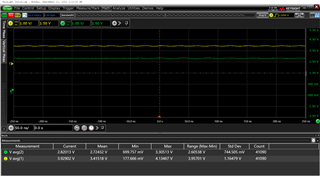Thanks,

João Rego

• Hi João,

Thank you for providing this information. I am still confused as to the last waveform you attached. I would expect the green waveform to be 2V if you are supplying the resistor divider 3V. And are you providing the opamp 5V or 4V in this waveform? The green waveform shows 2.75V so if you are supplying the opamp 4V, you will still be exceeding the common-mode input range. If you are able to attach a waveform with the op amp supply of 5V and resistor divider with 3V applied, that would be very helpful.

Also, when you measure with the 50ohm probe, can you confirm that you have an isolation resistor or some kind of terminated cabling on the output.

Thanks,

Nick

• Hi Nick,

The waveforms before are with a 5V Op Amp supply and 3V applied to the resistor divider. The IN+ input should be 2V, however, the Op Amp supply has an influence on its voltage inputs.

Now, I supplied the Op Amp with 4V and used 3V at the resistor divider (expected voltage at IN+ = 2V). But, you can see in the waveforms below that the IN+ is 2,5V (green waveform) and the 2nd stage output is 2,9V (yellow waveform).Then, I supplied the Op Amp with 5V and used 3V at the resistor divider (expected voltage at IN+ = 2V). But, the IN+ is now 2,8V (green waveform) and the 2nd stage output is 3,9V (yellow waveform). As you can see, the IN+ signal voltage changed with the Op Amp supply.I was expecting that the resistor divider could impose the IN+ voltage and then the 2nd stage output would have the same voltage as its inputs. However, this doesn't happen. Is the Op Amp being saturated at the V+ supply? Is the difference between the two signals due to the Vos*Gain?

I noticed that if I supply the Op Amp with 4V and connect directly a DC 2V voltage from the Power Supply, the circuit gets unstable. The waveforms below are: yellow is the 2nd stage output and in green is the IN+ voltage. In this case, the IN+ is 2V as expected.One note: the resistor divider is connected outside the PCB while the 1nF (connected to the IN+ pin) is on the PCB but not close to the IN+ pins. Is this an issue? I also read this on the datasheet: "Excess capacitance on an amplifiers input pin can reduce phase margin causing instability". Should I remove the capacitor at the IN+ pins? I placed it because the voltage coming from the resistor divider had some noise. You can see in the image below what I am referring to.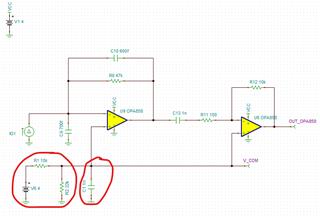When I measured with a 50Ohm probe, I had an isolation resistor of 47Ohm.

Thanks,

João Rego

• Hello João,

Thank you for providing detailed analysis. I will provide support since Nick is out off office.

This shouldn't cause an issue; it would be a concern at the IN- pin due to the feedback resistance. You are correct that the voltage bias should be consistent and you may be saturating the amplifier. But, you have the high pass filter at the second stage which is why the DC component will be removed from the first stage.

When you provide a signal from the photodiode and a reference DC voltage of 2V at IN+, what is your DC value at the output of the first stage and second stage?

Best Regards,
Sima

• Hello Sima,

I decided to remove the capacitor at the IN+ input pins, change the gain to 10 and place a series resistor of 150Ohm at the 2nd stage output. Below, is my current circuit. The photodiode is in parallel with C9 (0.7nF).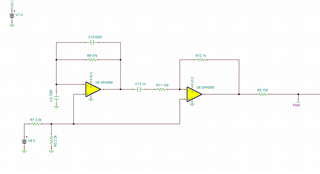Then, I measured the IN+ signal (yellow) and the output with a 50 Ohm probe (pink). As you can see in the images below, the IN+ DC voltage is what is expected (2V) and the 2nd stage output is oscillating around 2V too (the voltage measure at the oscilloscope is 1/4 of the 2nd stage output voltage due to the resistor divider 50/(50+150)).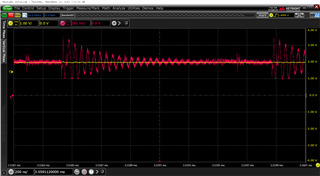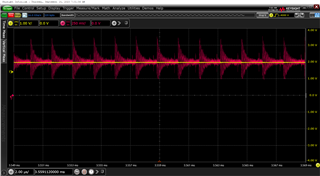Then, I probed with a 10:1 10Mohm probe, and now both signals oscillated. You can see in the images below the IN+ signal in yellow and the 2nd stage output in green.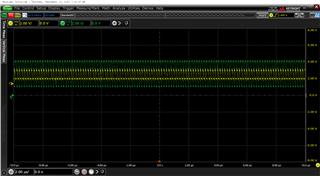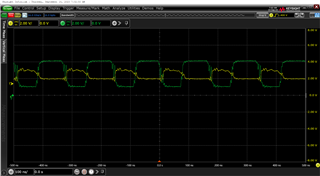I used the 50 Ohm probe again but supplied the APD with a different voltage. Now, the 2nd stage oscillation is different than what we saw before (it has an oscillation around 4V, the maximum output voltage with a 5V supply). Before, I used the TPS61391 to generate the APD voltage and, in fact, that oscillation was similar to the output ripple of that IC PCB.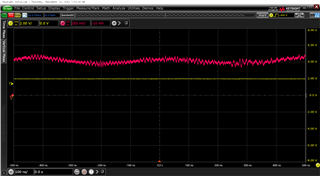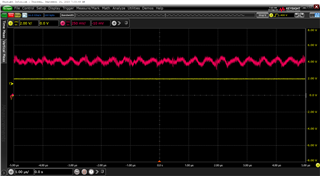Answering your question, when I don't have the capacitor at the IN+ input, the DC voltage is the voltage expected from the resistor divider. However, the 2nd stage output is oscillating. When I use the capacitor at the IN+ input (1nF), the 2nd stage output appears to be stable, however, the IN+ pin is not the expected DC voltage from the resistor divider and the 2nd stage output has a high offset.

I believe the problem can be the layout since the planes under the output weren't cut and the APD supply voltage is the left power plane at layer 3. Is this enough to make the circuit unstable?

Layer 1: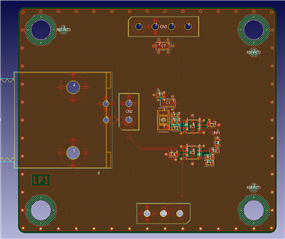Layer 2: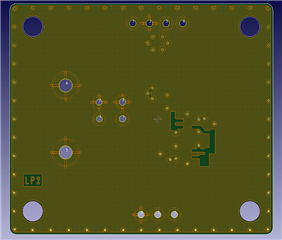Layer 3: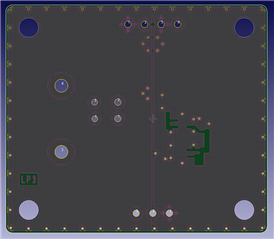Thanks,

João Rego

• Hi João,

These are very interesting phenomena you are experiencing, and we thank you for the detailed responses. I was able to run a stability analysis on the device with the Rf resistor of 1kohms like the circuit you highlighted above, and the phase margin is well above the 45-degree margin we recommend. The TINA file is attached and can be found below.

One debugging step that is recommended is to isolate the two stages and run them separately to test for oscillations. By removing the C13 (TINA schematic) you can separate the two stages. For the second stage, you could try working the board to run an arbitrary AC signal into the left side of R11. This will confirm that the first stage is truly stable and also confirm the second stage is the unstable stage not caused by the first stage.

Another suggestion if possible is to try putting the 1nF capacitor on the left side of R1 (TINA schematic). We do not recommend placing a capacitor directly on any input pin as the capacitor could cause stability issues as its interacting directly with the input stage. The R1 3.3kohm resistor between the capacitor and noninverting input pin will isolate the capacitance. Although it is interesting what you are seeing with the addition of this capacitor, the bigger issue might be that one or both stages are unstable, and the capacitor masks the issue but does not necessarily fix the issue.

We also looked over the layout and there is nothing that is standing out. We recommend cutting out the planes surrounding the feedback pin and the inverting input, any plane at the output pin should not have too much affect. It is hard to see exactly if all the layers are removed in the snippets, but it is recommended to minimize any parasitics. I did see you had an Riso (R3 in the layout snippets) at the input stage, and although it is highlighted in the datasheet, we have seen this Riso cause issues in some circuits. We tend to recommend not having this isolation resistor. Was this the intent of R3, if so, it would be worth setting R3 to 0ohms for future tests.

Best Regards,

Ignacio

2577.OPA858stability_E2E.TSC

• Hi Ignacio,

As you suggested, I isolate the two stages and test them individually. I applied a sine wave of 1MHz with 200mV peak-to-peak and 2V of offset at the resistor R11 (100Ohm on the TINA schematic). This waveform is the blue on the scope (it has an AC coupling). Then, I measured the IN+ input (that comes from the resistor divider) with the green probe and the Op Amp output (yellow waveform). As you can see, the IN+ DC voltage is 2V and the Op Amp output has a gain of -10. Although I thought the problem was the 2nd stage, these results show that the circuit is stable.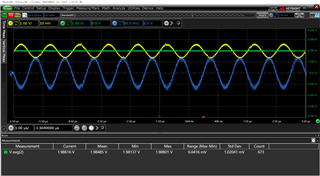So, I tested only the 1st stage (as I did in the first post of this forum). Measuring the output of the TIA without an isolation resistor gives an unstable circuit. The green waveform is the IN+ input and the yellow one is the 1st stage output. After I connected the isolation resistor of 10kOhm, the circuit became stable.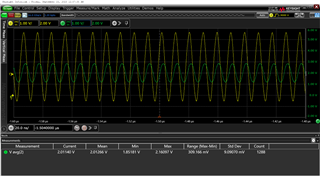I remember that although the simulation gave a phase margin above 45 degrees for an isolation resistor of 1kOhm, in reality, it didn't work. So, I removed the isolation resistor between the APD and the IN- input (as you suggested). However, the TIA was still unstable.

Placing the 1nF capacitor before the R1 3.3kOhm, makes the IN+ signal unstable. Below you can see the waveforms for the IN+ signal (green waveform) and the 2nd stage output with a 50Ohm probe (pink waveform). Basically, the Op Amp is saturated. This test was done with both stages.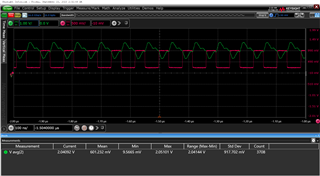Regarding the layout, all the planes below the inverting input and the feedback were cut. Only the planes below the 2nd stage output weren't cut. The planes below this output are the GND on the 2nd layer and the APD voltage on the 3rd layer. This APD voltage is generated through the TPS61391 and two charge pump stages. The ripple from this APD voltage is the ripple that appears at the output with the 50Ohm probe.

In conclusion, the 1st stage only works when I place an isolation resistor of 10kOhm between its output and the 10MOhm probe. The 2nd stage works well when alone. When I use both stages, the 50Ohm probe makes the output oscillate (IN+ is a fixed DC voltage) and the 10MOhm probe makes both the output and the IN+ oscillate. I noticed that if I connected a 10kOhm isolation resistor between the output and the 10MOhm probe, the circuit became stable.

Best regards,

João Rgeo

• Hi João,

For the second stage, does the output still show signs of instability or is it due to a non-ideal input signal? Could you try putting the device in a gain of say 20 V/V so changing the gain resistor and adjusting the input signal accordingly. Hopefully the ripple goes away in the output signal. As for the first stage, there could be a number of reasons why it is behaving this way. I would like to confirm the PD capacitance is being captured in the simulations appropriately. I understand the bandwidth requirement is around 5.6MHz, is the capacitance of the APD .7pF?

Best Regards,

Ignacio

• Hi Ignacio,

What do you mean by a non-ideal input signal? When I applied a wave signal from a function generator at the resistor connected to the IN- input of the Op Amp, the signal was amplified by the gain of -10V/V at the output. I believe the ripple at the output signal is due to the ripple from the function generator.

Yes, the APD capacitance is 0.7pF when supplied with a voltage above +/-70V. The feedback components give a bandwidth of 5.6MHz, as you said.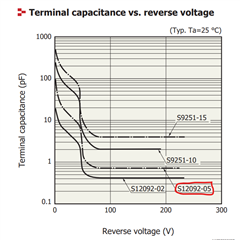Basically, when I use the Op Amps individually, only the 1st stage is unstable with a probe of 10MOhm (when measured with a probe of 50Ohm it is stable). When I use both stages, the system is unstable with a probe of 10MOhm. With this probe, both the output and the signal offset (IN+) are oscillating.

The 50Ohm probe makes the system stable. However, the output has a lot of ripple. Below you can see the following waveforms: in yellow is the APD supply with an AC coupling, in green is the reference voltage (IN+ input) and in pink is the output voltage. It is noticeable that the highest peak on the output voltage coincides with the highest peak on the APD supply.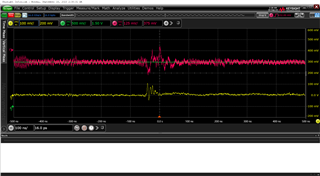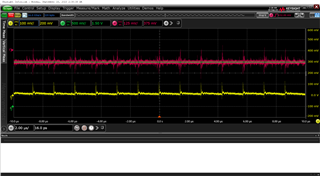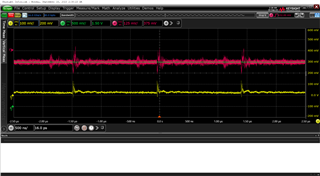Thanks.

Best regards,

João Rego

• Hi João,

I apologize for the confusion I was wondering if the ripple was due to the device or if the input signal had this ripple, that is what I meant by a non-ideal input signal. Small level oscillations sometimes occur due to instability which is why I was questioning if the second stage was truly stable. This is why the suggestion of increasing the gain was mentioned, but if it is due to the input signal then the second stage seems okay. When measuring the two stages, is there a way to directly measure the output into an oscilloscope instead of probing the signal? The probes seem to be altering the circuit, therefore ruling out any influence the probes have on the circuit would be ideal.

As a quick experiment, would it be possible to change the Cf capacitor to a smaller value than the 0.6pF on the board? This will change your bandwidth but for now the goal is to see if this helps stabilize the first stage.

Best Regards,

Ignacio

• Hi Ignacio,

What do you mean by measuring the output into the oscilloscope? Maybe I haven't used the correct terms. When I say that I measured with the 50Ohm probe, basically I was using a BNC cable that was directly connected to an oscilloscope, with a 50Ohm input. Is this what you refer to as measuring the output into the oscilloscope?

I noticed that my problems with the input capacitor at the non-inverting input were because this capacitor was too far from the Op Amps non-inverting input pins. As soon as I placed these capacitors as close as possible to the non-inverting pins, the ripple was attenuated and the output signal had the DC voltage from IN+. You can see in the image below the IN+ voltage (green) and the 2nd stage output voltage (pink) when it received a signal from the photodiode. Is it possible to reduce the ripple even more?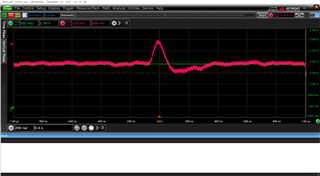This ripple has a peak-to-peak of 20mV. Although, sometimes this ripple increases to 60mV without any apparent reason. Also, if I zoom the ripple, a small oscillation of around 2GHz appears.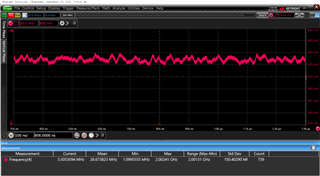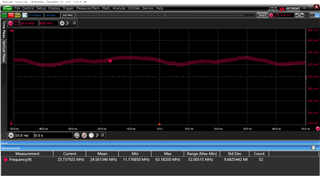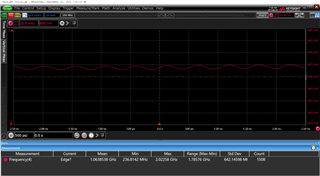Below is the current circuit.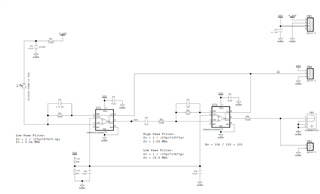Regarding the feedback capacitor, I will try to reduce it and see what happens.

Best regards,

João Rego

• Hi João,

You are correct, having a BNC cable that connects directly to the oscilloscope is what Ignacio was referring as it reduces the effects of extra capacitance in the circuit that probing introduces. From the information you have provided, it seems like the second stage may not be the issue as when you isolated the two stages and applied a signal to the second stage, you said that the output was stable.

With the two stages isolated, are you able to provide a waveform of the output of the first stage where you measure the output directly connected to the oscilloscope and not with a probe? This would rule out the influence the probes have on the circuit.

Also, it would be great to test the isolated first stage with the 600fF feedback capacitor and also a reduced feedback capacitor (something like 200fF). The reason for checking the reduced feedback capacitor has to do with having a gain that is large enough since the OPA858 is a decompensated amplifier that is 7V/V gain stable. Having the feedback capacitor at 600fF may be lowering the gain enough to leave the amplifier in an unstable region.

Thanks,

Nick

• Hi Nick,

I tested the 1st stage individually and measured it directly into the oscilloscope (50Ohm input). As you can see in the image below, the transimpedance amplifier was able to output a signal from the photodiode.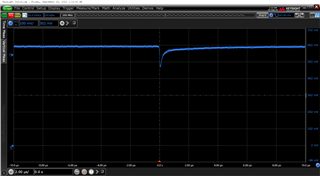Then, I took a closer look at the oscillation, and I saw the same oscillation of around 2GHz, as we have seen when the two stages were connected. This might be due to the feedback capacitor of 600fF since considering a feedback capacitor of 800fF (600fF + 200fF from parasitic) it gives a phase margin of 43 degrees at simulation.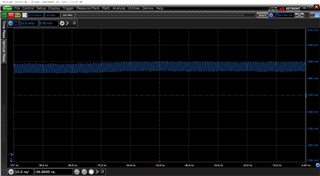As you suggested, I might reduce the feedback capacitor. Since I didn't have any capacitor below 600fF at the moment, I experimented to remove the feedback capacitor and didn't place any capacitor (just having the feedback resistor). As you can see in the image below, the oscillation of 2GHz was gone.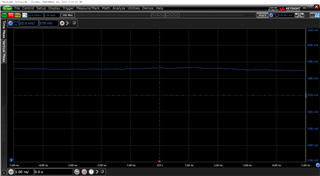For the following circuits, I will consider a capacitor around 200fF and 400fF or I will think about decreasing the resistor gain for the 1st stage.

Regarding the 2nd stage, although I have said previously that the circuit was stable, in reality, it has oscillations. I do not see the root of this instability since the stability simulation gave a stable circuit. Do you have any suggestions?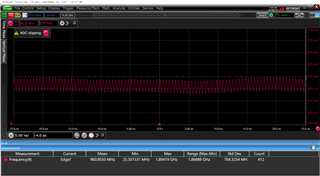Thank you.

Best regards,

João Rego

• Hi João,

As you saw, removing the feedback capacitor and letting the parasitic capacitance effectively be the feedback capacitor so it is a smaller value stabilized the first stage and removed the oscillations as expected. So I separated the second stage of your circuit and ran an AC analysis. The Bode plot shows that you are not achieving a bandpass filter with your current configuration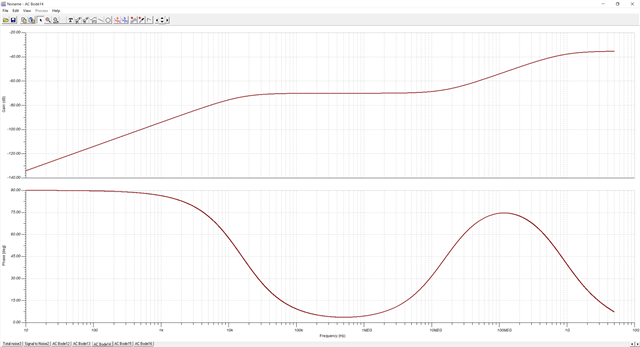I experimented with your filter and if I decrease the feedback capacitor to make a wider passband or if you decrease the gain by adjusting the feedback resistor, then the Bode plot shows a bandpass filter response. I suspect that the oscillations were appearing because the filter was not behaving properly.

My recommendation is to either use the TI Filter Design Tool to help design a filter that will meet your requirements or adjust the highpass and/or lowpass filters in your current configuration. A few things to note:

• Be careful adjusting the resistor and capacitor values as that could affect the stability of your circuit
• The passband bandwidth you are looking to achieve is considerably narrow; you may need to increase the passband bandwidth or look at a different filtering topology (which the TI Filter Design Tool should be able to help with)
• Hi Nick,

How did you run the AC analysis? I am asking because I got different results.

For the circuit below, which is the 2nd stage isolated, I have got the bandpass filter between 1MHz and 10MHz with a gain of 40dB. This is what I have designed.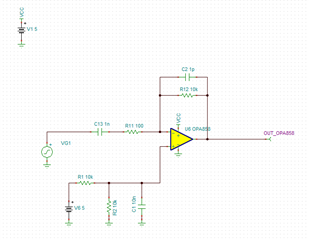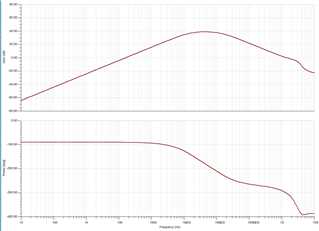Meanwhile, I am going to take a look at the TI Filter Design Tool in order to help me in the design of a filter.

Thanks.

Best regards,

João Rego

2475.OPA858_AC.TSC

• Hi João,

I ran an AC analysis again today to check the results I got and I am seeing the same results as you. I must have had something drawn incorrect so I apologize for that.

I am working on understanding why you are seeing oscillations and I look to provide you some information no later than end of day on Wednesday. I appreciate your patience.

Thanks,

Nick

• Hi Nick,

I have done some tests in the lab, but I don't know if it will help you.

With both stages isolated, I compared their oscillations. The 1st stage, without the feedback capacitor, had no oscillation, as we have seen before. The 2nd stage has an oscillation of around 1.5GHz. This circuit was modified to have a feedback capacitor of 0.5pF. It still has a gain of 100.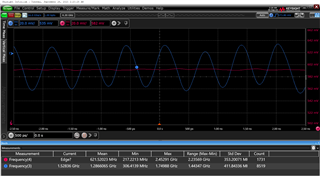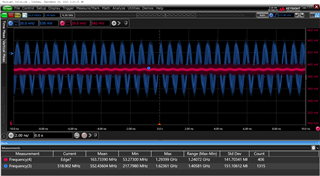Then, I compared the oscillations between the 2nd stage isolated and the complete circuit (1st stage + 2nd stage). The complete circuit has an oscillation of around 2.2GHz, higher than the 2nd stage isolated. The only difference between them is the output resistor (150 Ohm) position. The output resistor at the complete circuit (1st stage + 2nd stage) is closer to the Op Amp output.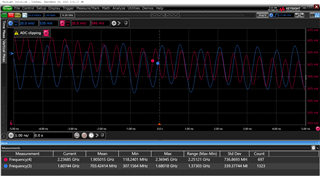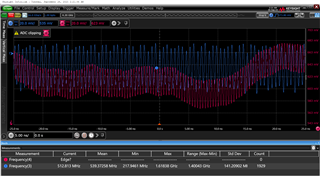Best regards,

João Rego

• Hi João,

Can you remove the feedback capacitor of the second stage, like you did for the first stage? I believe that the feedback capacitor plus parasitic PCB capacitance in conjunction are causing the second stage to become unstable.

Thanks,

Nick

• Hi Nick,

I have done that already and I got the same oscillation. Basically, before I changed the feedback capacitor from 1pF to 0.5pF, I tested the circuit without a feedback capacitor.

Thanks.

Best regards,

João Rego

• Hi João,

Thank you for confirming this. Could you try removing the 1nF capacitor and the feedback capacitor and changing the feedback resistor to 1k ohm so that the device is in a gain of 10? Also can you confirm what signal you are inputting (amplitude and frequency) and what bias voltage you are applying to the Vin+ terminal. I am trying to understand why you are getting an output around 600mV that I see in the plots you have provided.

Thanks,

Nick

• Hi Nick,

As you said, I removed the 1nF capacitor and changed the feedback resistor to 1kOhm. I had done this test in the past and remember that I also got oscillations. In the window below, you can see the output of the 2nd stage alone (without the 1st stage). It has oscillation with a frequency of 400MHz. The signal real amplitude is reduced by 4.7 due to the connection of a 180Ohm series resistor with the oscilloscope 50 Ohm input. If I place a capacitor on the feedback, I will still get the oscillation, however with a decrease in the oscillation amplitude.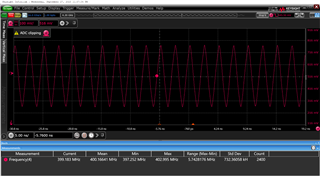There is no signal at the input (the input is open) so it was expected that the output would follow the non-inverting input which is a DC voltage of 2.5V.

This issue is taking too much of my time and since I already have another PCB (with a different Op Amp) working well, I will work with that. Nevertheless, I will still try to improve this circuit with a new layout and with a different Op Amp. Due to this reason, I would like to ask if there is any Op Amp that you can recommend to replace this OPA858.

Thank you for all the support.

Best regards,

João Rego

• Hi João,

I appreciate your patience while we work through trying to find a solution to the oscillation issues you are seeing, and I appreciate your very detailed responses. Based a previous response, I was under the assumption that when you isolated the second stage, you were applying a signal to the input from a signal generator. However, if you are leaving the input floating there is oscillation expected as leaving the input floating will make the op amp look like a unity gain op amp and the OPA858 is not stable in unity gain.

If you want to try testing the second stage again, you could either connect the second stage back to the first stage (since we have verified that the first stage is stable) or input a signal from a signal generator. I also ask that if you do test this again, to leave the 1nF and the feedback capacitor removed to determine if there are oscillations still with the amplifier in a basic inverting gain configuration.

I also completely understand wanting to pivot and try using a different op amp. You said that you are using a different op amp that is working well; are you able to provide details on what op amp that is? And I would be more than happy to provide a op amp recommendation to you, I just have a couple questions so that I can provide the best recommendation:

• I assume that you are looking for a single supply amplifier that runs at 5V based on how you are powering the OPA858. Is this correct?
• Can you provide the current output of the photodiode or how much you need to amplify the signal? Also what frequency is the photodiode signal?
• Are you looking for a recommendation for the second stage amplifier or are you looking for a different recommendation for the first stage TIA as well?

Thanks,

Nick

• Hi Nick,

So, I applied a signal from the signal generator to the 2nd stage, to test it individually. The input signal was being amplified by a gain of -10. However, the oscillation was still present with a frequency around GHz. You can see in the images below the output of the 2nd stage. In the past, I have already tested the 1st stage+2nd stage and I still got the oscillations.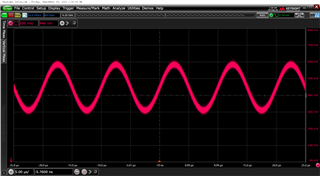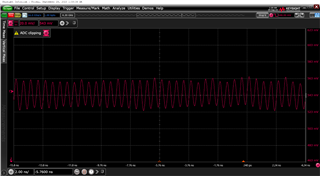The other circuit that I was referring to working well is using the OPA356 on all the stages. However, due to the bandwidth limitations, I have 2 inverting stages with a gain of -10 instead of just one with a gain of -100. The main limitation of this circuit is the higher values of noise, which were expected based on the spice simulation.

•  Yes, a single supply that runs up to 5V with low noise and a disabled pin.
• The photodiode current can be 1nA up to 500nA.
• At this moment, I am mainly focused on a recommendation for the 2nd stage (or, if needed, the use of 2 inverting stages as I did with the OPA356) since the 1st stage is working very well. However, if you have any suggestions for the 1stage, it would be welcome.

Thank you.

Best regards,

João Rego

• Hi João,

Thanks for providing your requirements. I think there are two amplifiers that would suit your application well.

I would recommend either the OPA859 which is a unity gain stable voltage feedback amplifier. Another option that may work is the OPA695 which is current feedback amplifier.

Please let me know if you have any questions about these devices.

Thanks,

Nick